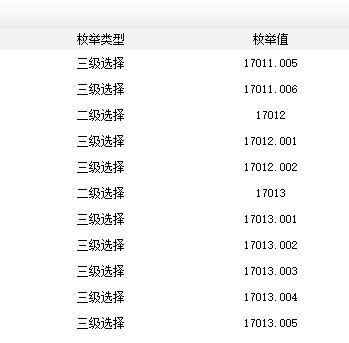# dedecms织梦自定义表单添加地区三级联动正确的修复方案

2、修改的文件：dede/templets/diy_field_edit.htm 大概在127行，很明显已经注释掉，我们把注释去掉，然后放到“多选框”下面。

 1 2 3 4 5 6 else if($fielddata=='addon') {$fields[$field] = " 其它附件"; } 在其 下面 添加如下代码  1 2 3 else if($fielddata=='stepselect')       {          $fields[$field] = $em_nativeplaces[GetEnumsTypes($fields[$field])['top']].$em_nativeplaces[GetEnumsTypes($fields[$field])['son']].$em_nativeplaces[GetEnumsTypes($fields[$field])['sun']]; } 打开 dede/diy_list.php文件，找到 else if ($action == 'list')
{

include_once DEDEINC.'/datalistcp.class.php';
在下面添加如下代码

include_once(DEDEDATA.'/enums/nativeplace.php');
include_once(DEDEINC."/enums.func.php");

/**
*  获取联动表单两级数据的父类与子类
*
* @access    public
* @param     string  $v * @return array */ function GetEnumsTypes($v)
{
$rearr['top'] =$rearr['son'] = $rearr['sun'] = 0; if($v==0) return $rearr; if($v%500==0) {
$rearr['top'] =$v;
}
else{

$rearr['son'] =$v;
$rearr['top'] =$v - ($v%500); } return$rearr;
}

/**
*  获取联动表单两级数据的父类与子类
*
* @access    public
* @param     string  $v * @return array */ function GetEnumsTypes($v)
{
$rearr['top'] =$rearr['son'] = $rearr['sun'] = 0; if($v==0) return $rearr; if($v%500==0) {
$rearr['top'] =$v;
}
else{
$rearr['sun'] =$v;
$rearr['son'] = intval($v);
$rearr['top'] =$v - ($v%500); } return$rearr;
}# 微信扫码购买修复包05-14231404-058014
01-3060
09-0258
01-201万+
05-307191
05-192488
11-0430
02-25705
11-0436
11-032992
05-20
09-18
01-171万+
08-2310万+